## Example Questions

2 Next →

### Example Question #382 : Plane Geometry

Which of the following cannot be the measure of an exterior angle of a regular polygon?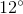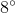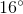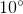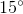Explanation:

If one exterior angle is taken at each vertex of any convex polygon, the sum of their measures is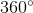. In a regular polygon - one with congruent sides and congruent interior angles, each exterior angle is congruent to one another. If the polygon hassides, each exterior angle has measure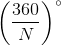.

Given the common measure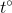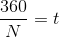Multiplying both sides by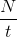: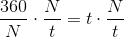and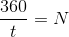Sinceis equal to a number of sides, it is a whole number. Thus, we are looking for a value ofwhich, when we divide 360 by it, yields a non-whole result. We see thatis the correct choice, since'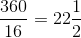A quick check confirms that 360 divided by 8, 10, 12, or 15 yields a whole result.

### Example Question #11 : Other Polygons

To the nearest whole degree, give the measure of each interior angle of a regular polygon with 17 sides.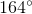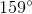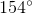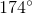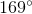Explanation:

The measure of each interior angle of an-sided polygon can be calculated using the formula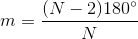Setting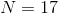: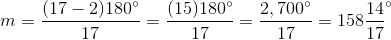The correct choice is therefore.

### Example Question #384 : Plane Geometry

Each interior angle of a regular polygon has measure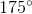. How many sides does the polygon have?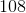Explanation:

The easiest way to work this is arguably to examine the exterior angles, each of which forms a linear pair with an interior angle. If an interior angle measures, then each exterior angle, which is supplementary to an interior angle, measures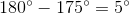The measures of the exterior angles of a polygon, one per vertex, total; in a regular polygon, they are congruent, so if there aresuch angles, each measures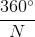. Since the number of vertices is equal to the number of sides, if we set this equal to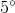and solve for, we will find the number of sides.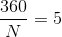Multiply both sides by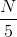: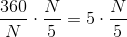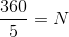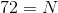The polygon has 72 vertices and, thus, 72 sides.

### Example Question #11 : How To Find An Angle In A Polygon

A regular polygon has a measure of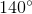for each of its internal angles.  How many sides does it have?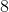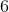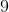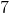Explanation:

To determine the measure of the angles of a regular polygon use:

Angle = (n – 2) x 180° / n

Thus, (n – 2) x 180° / n = 140°

180° n - 360° = 140° n

40° n = 360°

n = 360° / 40° = 9

2 Next →

### All SAT Math Resources